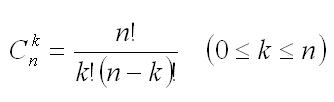favorite We need a little bit of your help to keep things running, click on this banner to learn more
Competitions

# Binomial coefficients 1

Let n be a non-negative integer. Let n! = 1 * 2 *... * n (0! = 1) andYou are given the numbers n and k. Calculate C(n,k).

#### Input

The first line contains the number of test cases t (t50). Each of the next t lines contains two integers n and k (0n < `264`, 0C(n,k) < `264`).

#### Output

Print t lines, each contains the value C(n,k) for corresponding test.

Time limit 1 second
Memory limit 128 MiB
Input example #1
```6
0 0
1 0
1 1
2 0
2 1
2 2
```
Output example #1
```1
1
1
1
2
1
```
Author Anton Lunyov